# Light Dependent Resistor (LDR)

### From DT Online

##### Description

The resistance of Light Dependent Resistors (LDR) decreases when light falls on them.

##### Features and Applications

A typical example is the ORP 12 type which will vary its resistance from about 2 Megohms in the dark to 100 ohms in normal room lighting.

LDRs are frequently used as sensors in light activated switch circuits, for example, to switch on lights in the evening when it starts to get dark.

A signal of 0.6V, or greater, to the BASE of the transistor "switches on" the transistor and allows a current to flow in the COLLECTOR / EMITTER circuit.Transistors also act as CURRENT AMPLIFIERS since a small base current (Ib) controls a much larger collector current (Ic). The amplification (GAIN - hfe) of a transistor, is given by the equation hfe = Ic/Ib and is typically several hundreds. (Note that the use of a variable resistance in the other half of the potential divider circuit allows the sensitivity of the circuit to be adjusted).

##### Light Sensor

Many "control" circuits are triggered by a signal which is produced by a "potential divider" network. The signal value depends upon the component values and the supply voltage:

Vs=V*R2/(R1+R2)

where:

• Vs is the signal value
• V is the supply voltage
• R1 is the resistance between the positive supply rail and the base of the transistor
• R2 is the resistance between the negative supply rail and the base of the transistor

An LDR decreases its resistance with an increase in light intensity. As light increases, therefore, the resistance of the LDR decreases and allows the voltage at the base of the transistor to move closer to that of the positive supply rail.

##### Dark Sensor

As with the Light Sensor, this circuit is triggered by a signal which is produced by a "potential divider" network and the signal value depends upon the component values and the supply voltage:

Vs=V*R2/(R1+R2)

where:

• Vs is the signal value
• V is the supply voltage
• R1 is the resistance between the positive supply rail and the base of the transistor
• R2 is the resistance between the negative supply rail and the base of the transistor

An LDR decreases its resistance with an increase in light intensity. In this position therefore, as light decreases the resistance of the LDR increases and allows the voltage at the base of the transistor to move closer to that of the positive supply rail.

Note:

• When choosing a transistor it is important to make sure that the gain is high enough for the required use and that the maximum current which it can handle is sufficient to drive the output device. e.g. BFY 51 gain > 40 , max Ic = 1 amp.
• If the max Ic value is exceeded, the transistor will probably "burn out".
• The transistor acts as a NOT gate (inverter) since a "high" at the base gives a "low" at the collector. (i.e. when the transistor switches on, the collector voltage decreases).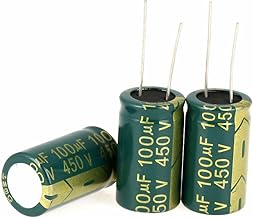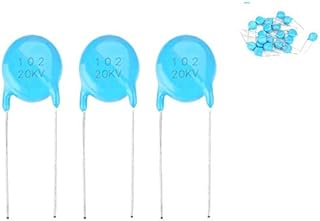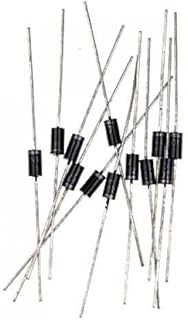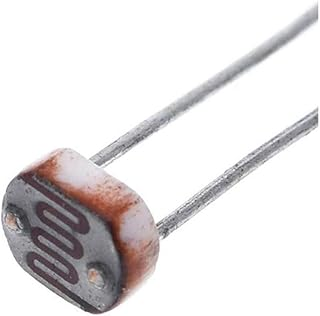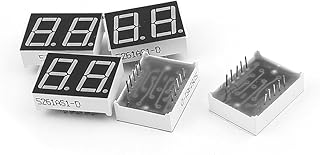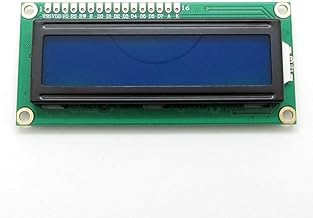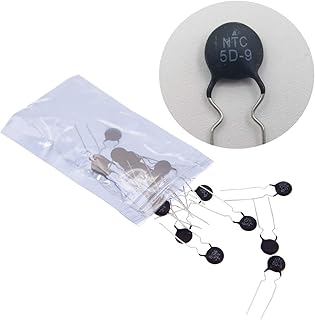1/4w Resistors PackElectrolytic CapacitorsCeramic Disc CapacitorsRectifier DiodesPhoto Light Sensitive Resistor (LDR)Light Emitting Diodes (LED)Common Cathode LED DisplayLCD Module DisplayThermistor Resistor